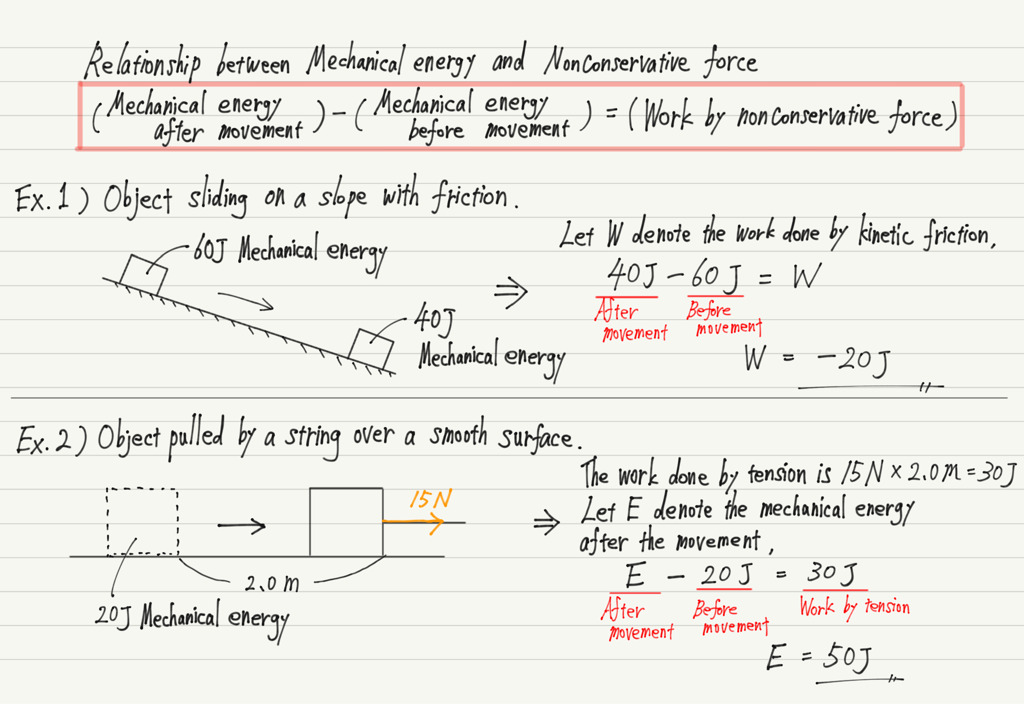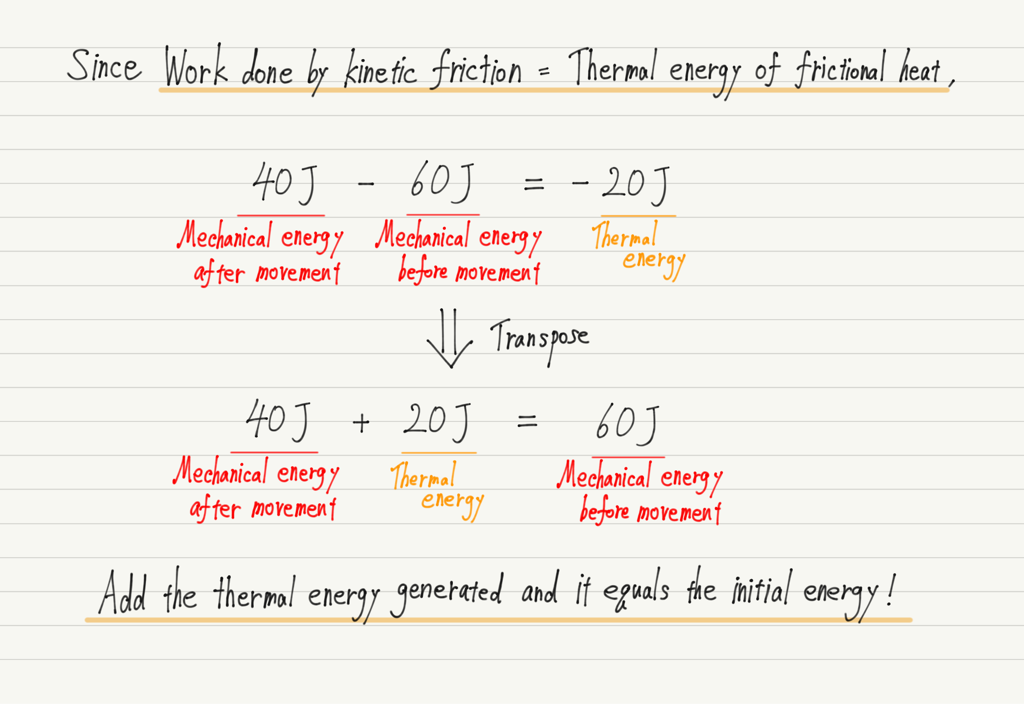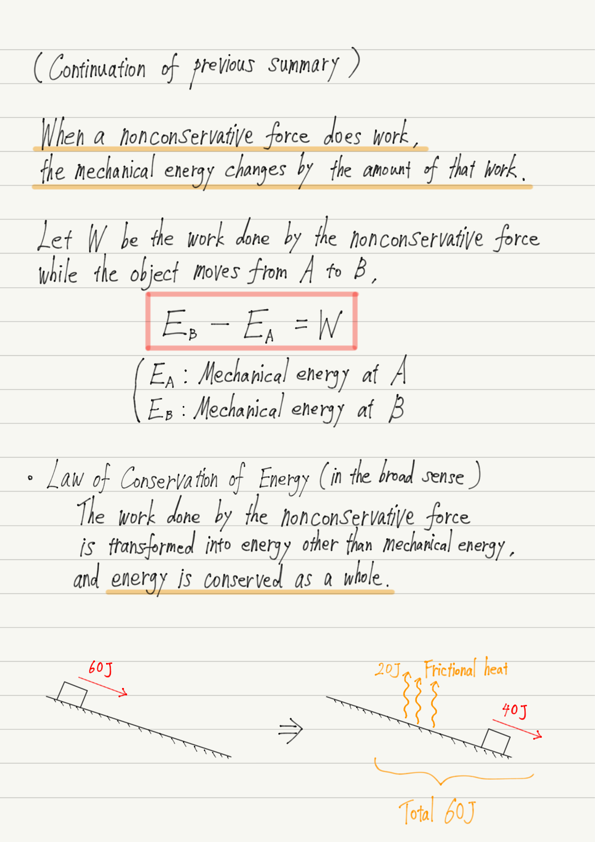# Mechanical Energy and Nonconservative Force

In the previous lecture, we learned about the law of conservation of mechanical energy.

https://www.yukimura-physics.com/entry/dyn-f29

The point was that mechanical energy is not always conserved, but only when a conservative force does the work.
This in turn means that mechanical energy is not conserved when nonconservative forces do work.

When we talk about mechanical energy, the “conservative case” tends to take the lead, but let’s study the “nonconservative case” as well.

## Equation for “change” of mechanical energy

In the case of conservation of mechanical energy, we calculated the mechanical energy at two points and connected them by equality.
However, in the nonconservative case, they cannot be connected by equality.
That is, it cannot be calculated!

But it’s not too early to give up.
The fact that it is not conserved means that the mechanical energy is increasing or decreasing, right?

Don’t you think it can be calculated if you know how much it will increase (or decrease)?

If nonconservative forces do the work, it looks like we can formulate a “Equation for change of mechanical energy” instead of a “Equation for conservation of mechanical energy”!

## Only work can change energy

As we have repeated many times before, there is a close relationship between energy and work.

If an object does work, its energy is reduced.
If an object is worked, its energy is increased.

This is also true in the case of mechanical energy.
The reason why mechanical energy is not conserved in this case is, of course, the nonconservative force.

That means the mechanical energy changes by the amount of work done by the nonconservative force!## Energy is conserved as a whole

In Ex.1 above, the important point is that the mechanical energy is reduced by the work done by the kinetic friction, but has the reduced energy disappeared?

In fact, it is known that all work done by kinetic friction is converted into thermal energy (heat of friction).
With this in mind, the equation in Ex.1 can be reviewed as follows.Mechanical energy is not conserved because of friction, but if we read the work done by friction as thermal energy, the total energy, including heat, is conserved!

Energy comes in many forms. Mechanical energy, thermal energy, electrical energy, light energy, chemical energy, nuclear energy,…

Even in cases such as mechanical energy, which does not appear to be conserved when viewed in isolation, the reduced amount is transformed into other forms of energy, and energy as a whole is conserved.

“Mechanical energy” may not be conserved, but “Energy (in the broad sense)” is always conserved.

## Summary of this lecture## Next Time

This concludes the mechanics section of the Basic Physics.
After this, we will move on to the Advanced Physics.
Since we will be making full use of the content learned in the Basic Physics, please review all the content you have studied so far before proceeding.

https://www.yukimura-physics.com/entry/dyn01

error: Content is protected !!